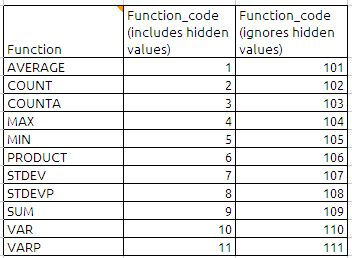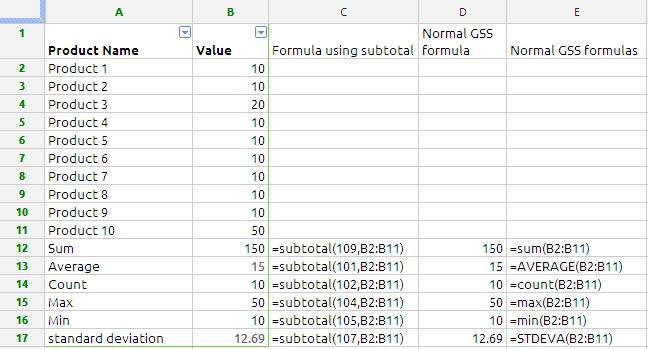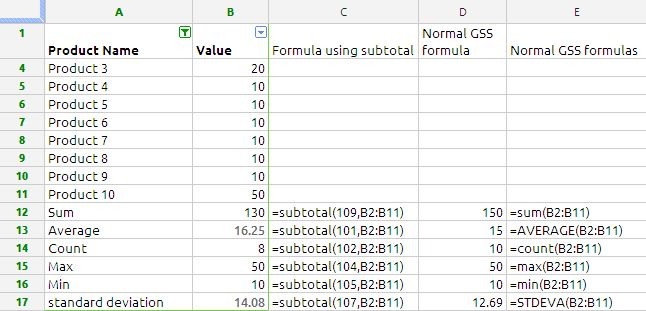## SUBTOTAL

Subtotal is great function specially when you using filter to your data. The syntax for the function is as follows:

SUBTOTAL(function_code, range_1, range_2, … range_30)
function_code: the subtotal function behave differently depending on the function_code it some time behave like counter,minimum, maximum, sum, average etc. depending on the data range. The complete code list is as follows:ex: if we selected the function_code as 9 then we will get the total of the given range including hidden values. where as if we selected the 109 the function ignores the hidden values in the range. this i will explain in the subsequent paras

range_1,range_2,…..range_30: A collection containing the values to be evaluated. data is a collection containing number, a string  or date/time values or. All values should be of the same type.

Now lets see subtotal function with an exampleThe data is from cell A1 to B11, we have formula using subtotal function from B12 to B17 and we have traditional formulas from cell D12 to D17 Google Docs Spread sheets. suppose we filter data and hidden Product1 and Product 2 the data is will be as below:the beauty of the Subtotal function is can be clearly seen in the above example. the subtotal formulas are working perfectly and our traditional formula have failed to give correct answers because the traditional formulas are taking the hidden ranges also.